# What is lever arm physics

## Lever¶

A lever is a rigid, usually rod-shaped body that can be rotated around a fixed axis (e.g. a rocker). Levers are used, for example, to lift a body with a large weight with a small force.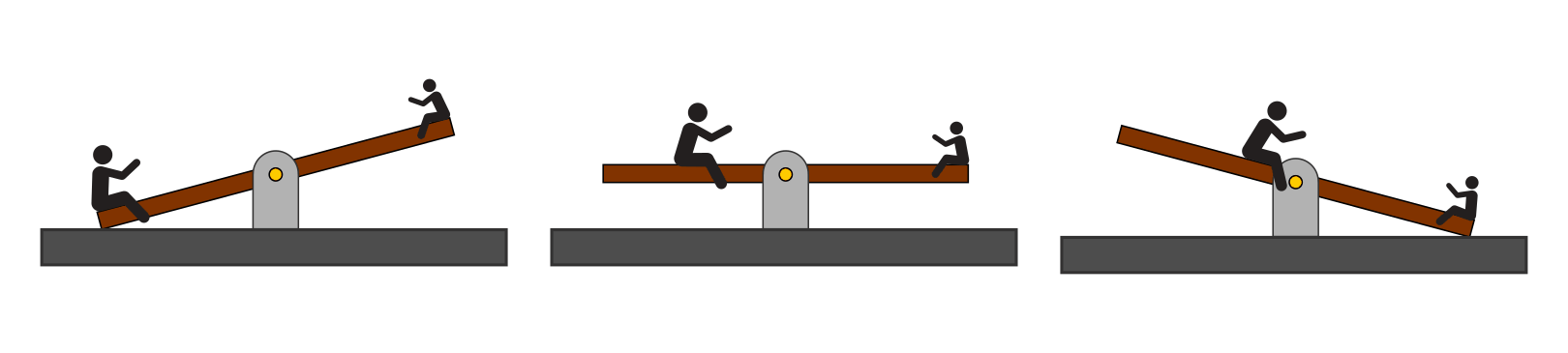The rocker as a (two-sided) lever: Not only the acting force, but also the distance to the axis of rotation is important.

The place where a force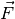acts on the lever is called the point of application of the force, the distance between its line of action and the axis of rotation becomes the force arm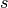called.

Two forces act on a lever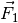and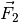so the lever is then in equilibrium when the product first forcewith her power arm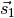equal to the product of the second forcewith her power arm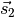is:

The above formula, which formally describes the equality of two torques, is also known as the lever law. It applies equally to all lever types.

### Double sided levers¶

On a two-sided lever, the points of application of the forces, viewed from the axis of rotation, are on different sides of the lever.

The lever is in balance when the torque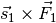on the left side of the lever the torque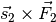on the right side of the lever.  If the acting torque is greater on one side than on the other, the lever rod executes a corresponding rotation around the axis of rotation.

### Unilateral levers¶

On a one-sided lever, the axis of rotation is at the edge of the lever rod, so that the points of application of all the forces acting, viewed from the axis of rotation, lie on the same side of the lever.

In order for an equilibrium to prevail on a one-sided lever, the sum of the counterclockwise torques must again be equal to the sum of the clockwise torques. To do this, the forces acting on a one-sided lever must point in opposite directions. 

A striking example of a one-sided lever is the human forearm. The elbow acts as the axis of rotation, the weight of the object to be carried acts downwards, the muscle strength of the biceps upwards. Since the biceps muscle engages the forearm many times closer to the axis of rotation than the weight to be carried, the force acting there must be correspondingly many times greater.

### Angle lever¶

A lever with an angled rod is called a bell crank. Depending on where the axis of rotation is located, an angle lever is like a one-sided or two-sided lever.

The law of leverage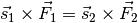also applies to an angle lever unchanged. If the left-hand torque is the same as the right-hand torque, there is a lever balance; otherwise a corresponding rotation about the axis of rotation occurs.

### Well gear and crank¶

A corrugated gear consists of (at least) two differently sized and interconnected wheels that sit firmly on an axle ("shaft"). The rims of the wheels can consist of a solid material or be connected to the axle via spokes.

In principle, a corrugated gear is also a lever , so that in the case of equilibrium, the general lever law applies again: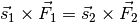The distances correspond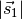and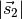the radii of the two wheels. In terms of amount, the force amplification that occurs when the outer wheel rotates in relation to the inner wheel is equal to the ratio of the radii of both wheels: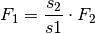A small force on the big wheel therefore results in a large force on the small wheel. A crank works according to the same principle. This can be imagined as a single, massive spoke of a corrugated gear.

Example:

• A crank with a radius of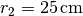becomes with a force of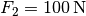driven. The force that this creates on a drive wheel with a radius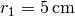acts, is therefore: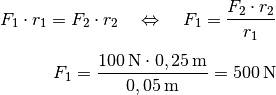In this case, too, a small force on the long pedal rod causes a large force on the small drive wheel.

Remarks: